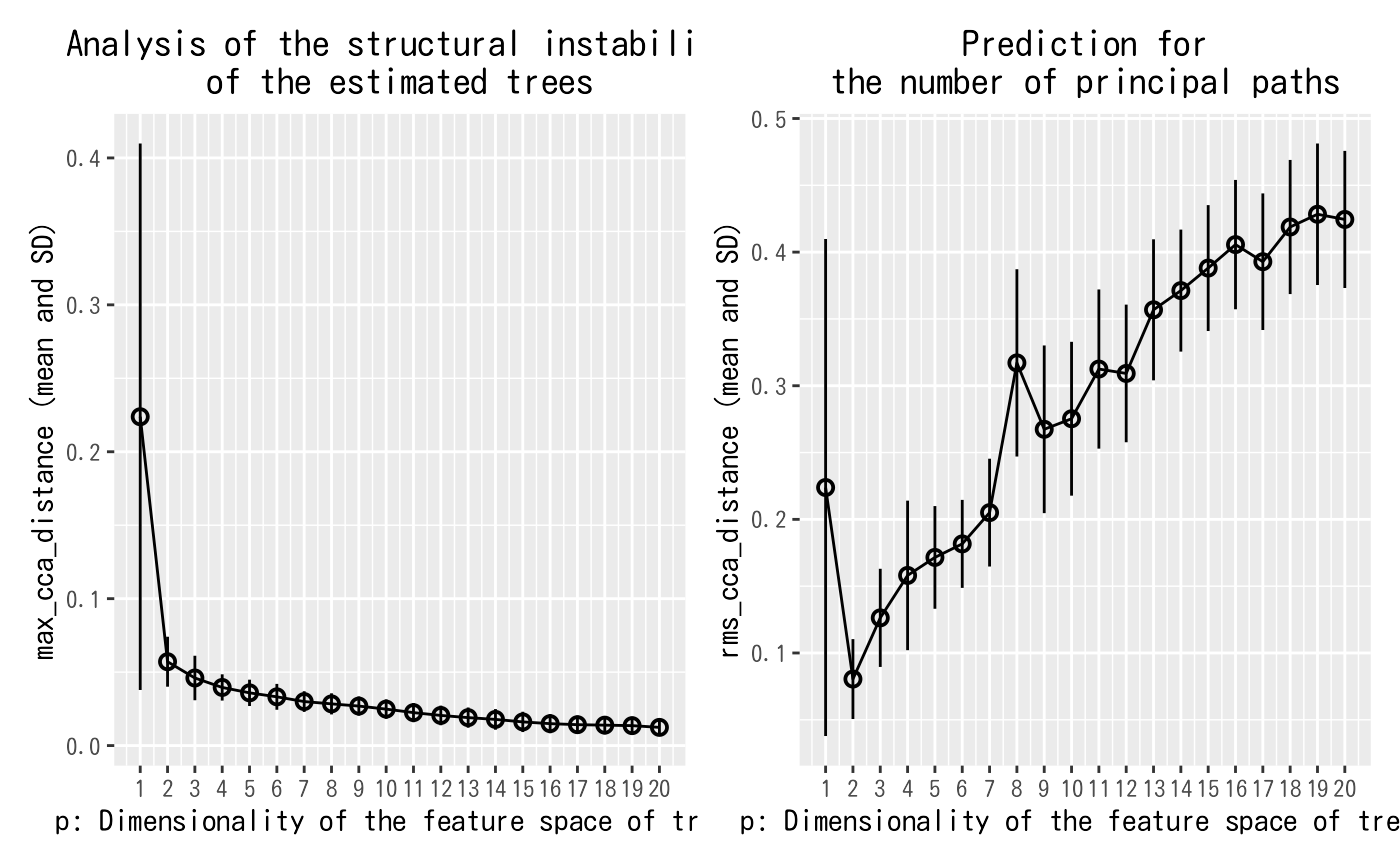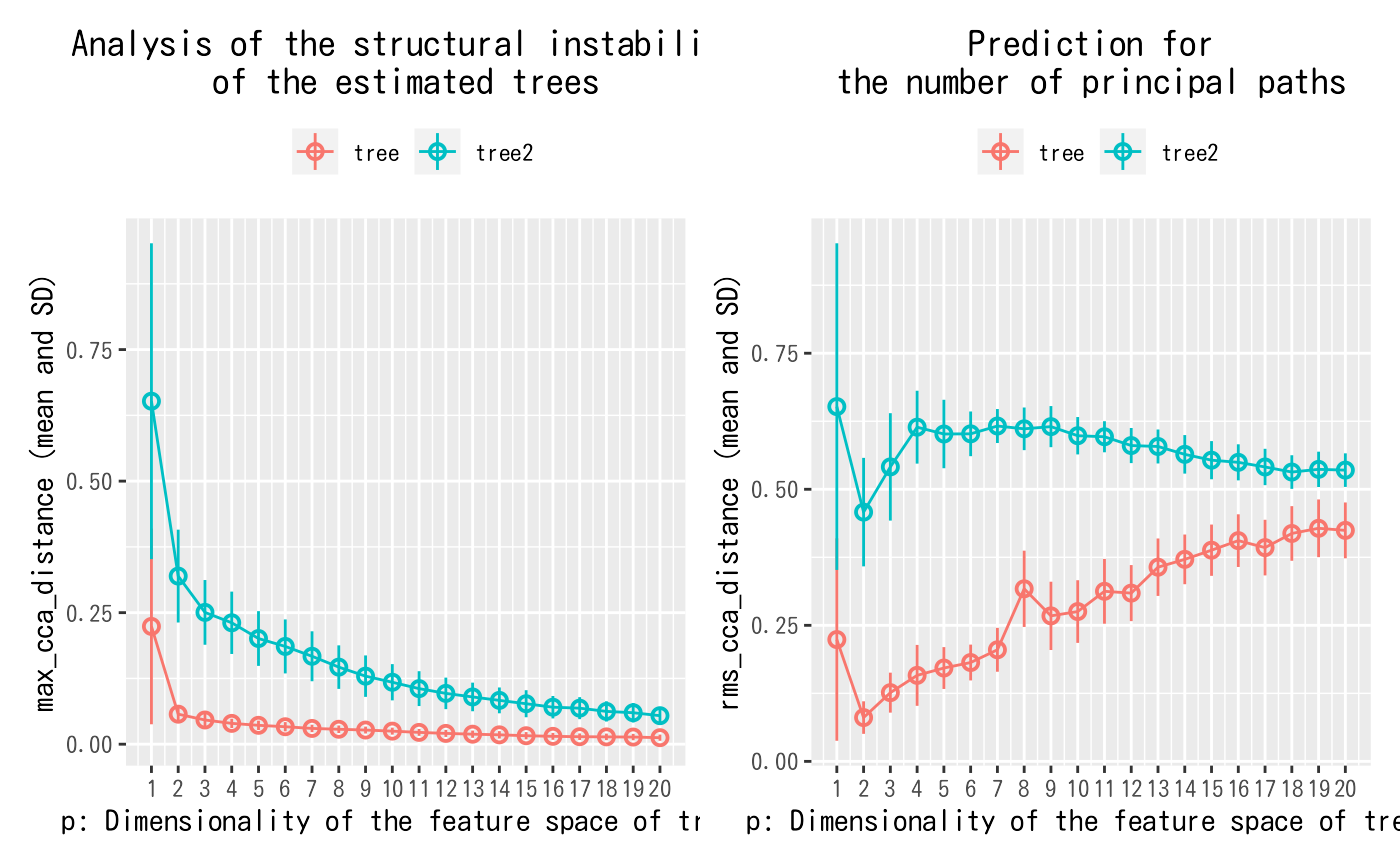Plot estimate results to get insight.

# S3 method for treefit
plot(x, ...)

## Arguments

x

The estimated result by treefit() to be visualized.

...

The more estimated results to be visualized together or other graphical parameters.

## Value

A plot object as a ggplot object. It plots the given one or more estimated results to get insights from one or more treefit() results.

## Examples

# \dontrun{
# Generate a tree data.
tree <- treefit::generate_2d_n_arms_star_data(200, 3, 0.1)
# Estimate the goodness-of-fit between tree models and the tree data.
fit <- treefit::treefit(list(expression=tree), "tree")
# Visualize the estimated result.
plot(fit)# You can mix multiple estimated results by adding "name" column.
tree2 <- treefit::generate_2d_n_arms_star_data(200, 3, 0.9)
fit2 <- treefit::treefit(list(expression=tree2), "tree2")
plot(fit, fit2)# }